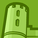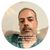# QlikView App Dev

Discussion Board for collaboration related to QlikView App Development.

Announcements
Skip the ticket, Chat with Qlik Support instead for instant assistance.
cancel
Showing results for
Did you mean:Not applicable

## Error in expression : ')' expected

Hi does anyone know what I did wrong ?

if(\$(xxx),'130',

if(\$(xxx),'140',

if(\$(xxx),'20',

if(\$(xxx),'30',

if(\$(xxx),'0','100')))))

Anthony

17 RepliesPartner - Contributor III

Hi ,

Is the variable created correctly . check in variable overview whether it is created or not .Master III

Try like this as you need to check for the value of the variable..If it returns a -1

=if(\$(TickXOIP)=-1,'130',

if(\$(TickInternational)=-1,'140',

if(\$(fils)=-1,'20',

if(\$(NBDGPM)=-1,'30',

if(\$(ClosStream)=-1,'100')))))Master

Hi,

Check the variable expressions, u might not closed  this bracket ')'Specialist II

\$(XXX) is a variable right try to put single quotes and check

if('\$(xxx)','130',

if('\$(xxx)','140',

if('\$(xxx)','20',

if('\$(xxx)','30',

if('\$(xxx)','0','100')))))

or

if('\$(TickXOIP)'='130','130',

if('\$(TickInternational)'='140','140',

if('\$(fils)'='20','20',

if('\$(NBDGPM)'='30','30',

if('\$(ClosStream)'='100','100'))))))Not applicable
Author

try like this

if('\$(TickXOIP)','130',

if('\$(TickInternational)','140',

if('\$(fils)','20',

if('\$(NBDGPM)','30',

if('\$(ClosStream)','100'))))))Not applicable
Author

Now I'm just trying to do that

if(\$(TickXOIP),'130%',

if(\$(TickInternational),'140%','other')

TickXOIP=(\$(ISUSCPilote) + \$(ActivesISTOIP) + \$(BTIPBTIC))

ISUSCPilote=count({<IXOIPIS={1}>} distinct PHT_IDTTIC)

ActivesISTOIP=sum(ISTOIP)

BTIPBTIC=count({<IBTICBTIP={1}>} distinct PHT_IDTTIC)

TickInternational=(\$(scope0) + \$(scope1) + \$(Scope2))

scope0=count({<SCOPE0={s0}>} distinct PHT_IDTTIC)

scope1=count({<SCOPE1={s1}>} distinct PHT_IDTTIC)

scope2=count({<ISCOPE2={1}>} distinct PHT_IDTTIC)

So i did that :

if(SCOPE0='s0' or SCOPE1='s1' or ISCOPE2='1' ,'140%','other') it's working well

if i'm trying that

if(IXOIPIS='1' or ISTOIP='1' or IBTICBTIP='1' ,'130%','other') it's working also

but when i'm doing this it's doesn't works anymore

if(SCOPE0='s0' or SCOPE1='s1' or ISCOPE2='1' ,'140%',
if(IXOIPIS='1' or ISTOIP='1' or IBTICBTIP='1' ,'130%','other'))

any idea ?

AnthonyMVP

Can you share what output you get for the expression you said not workin, as we don't know what do you mean by it doesn't work any more.

Also can you put these condition separately in separate text box -

if(SCOPE0='s0' or SCOPE1='s1' or ISCOPE2='1' ,'140%') in one text box and

if(IXOIPIS='1' or ISTOIP='1' or IBTICBTIP='1' ,'130%','other') in other text box

and share the results.Anonymous
Not applicable
Author

THANK YOU!! I couldn't figure out why my script was throwing this error. I had comments in a pretty lengthy expression to keep things straight that was causing my error- Not sure if this was the original posters problem, but definitely helped me. Thank you!Community Browser